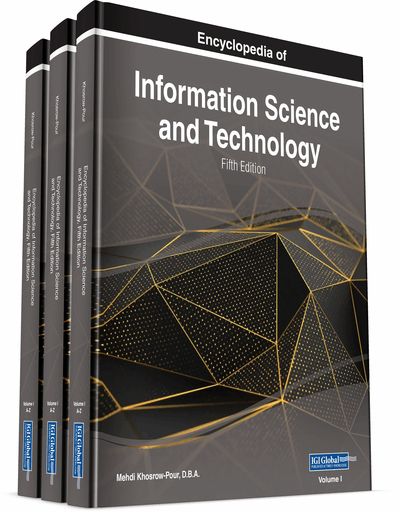# Sense and Boundaries of Computer Simulations

DOI: 10.4018/978-1-7998-3479-3.ch012
OnDemand:
(Individual Chapters)
Available
\$29.50
No Current Special Offers

## Abstract

Computers are widely used in physics and other natural sciences to simulate physical phenomena. Thus, people routinely use computers to model many and different physical systems. In addition, computers have been used to solve difficult problems by simulating successful practices employed by living organism. However, it seems not everything can be simulated, that is, there are phenomena that are characterized as non-computable. Most of them can be simulated by quantum computers but for some even these computers are not adequate. The authors examine why this happens, how it can be solved, and whether the “ultimate” goal of simulating the whole universe is feasible.
Chapter Preview
Top

## Introduction

It is generally well established that a good many phenomena of everyday life are quite complicated to be described in a simple, concise and quantitative way. Many examples can spring to mind; from the motion of an object in the Earth’s atmosphere to the (long term) weather forecasting, or from bacteria growing experiments in a Petri plate to space craft missions, etc. Indeed, almost all the physical (real) systems, say from natural sciences (e.g., physics, chemistry, biology, geology etc.) to applied sciences (e.g., engineering, economics etc.), and from health sciences to humanities are susceptible to a – more of less – multi parametric and complex prototype.

The aforementioned complexity is due to the following reason: The magnitude of the system under consideration is beyond any reasonable reach and completely unlikely to be reproduced in the laboratory for study

• a)

either at a local level; indeed, for example, although it is quite simple to deal with the motion of a gas molecule, it is extremely difficult for a human to do the same with the motion/behaviour of an enormous amount of molecules interacting with each other;

• b)

or at a global level, for example, the weather forecast in meteorology, the galaxy collisions in Relativistic Astrophysics, etc., for obvious reasons.

Conceptually, case (a) is by default attacked by implementing statistical methods, while case (b) is attacked by multi parametric analytical systems. Nevertheless, both cases are dealt with the use of computers. In other words, the previously mentioned multi parametric, complex prototype is realised as a (imperative) computer code of a functional nature: some input is given and an output is expected (thus the imperative character); also same inputs produce same results (hence the functional character). This computer code incarnates the term “computer simulation” [see (Osais, 2017) for an overview of the programming aspects of simulation].

Definition 1. A computer simulation is a computer program that represents the dynamic responses of one system by the behaviour of another system modelled after it.

Top

## Background

Computers can be used to simulate physical systems but is the use of computers (and thus the need for computer simulations) something unavoidable? The answer to this question is affirmative.

Indeed for the case (a), general theorems from Statistics dictate that the larger the magnitude of the prototype the more accurate and with less deviation is the forecast for the behaviour of the system. Within this sector of this type of problems the need for the use of random numbers (expressing an arbitrary possible state of the system) is a sine qua non. Of course, it is practically impossible for a human to produce massively random numbers and thus to emulate a plausible (physical) state of the statistical system under consideration. At this point the computers enter the study; they can produce in (almost/relatively) no time huge quantities of arbitrary numbers, therefore they suffice for the description of the collection of physical states. Yet, there are two limitations here:

• On one hand, computers produce pseudo random numbers and not completely random ones. This has to do with the whole concept of what a human made machine is capable of. Modern techniques have been tried to smooth away this problem by implementing characteristics of the machine (like the temperature of the CPU or the “entropy” of the accumulated information etc.) which could be used to produce truly random numbers, but the very essence of the problem remains since in any case correlating functions are involved.

• On the other hand, computers do not cope well with real numbers, but only with integers. Thus, two real numbers which are supposed to be equal, do not have their difference equal to zero but rather equal to a given (very) small number. For instance, 0.99999 and 1 can be consider as equal (if the tolerance/accuracy is, say, 0.00001) or not –depending both on the hardware architecture and the software constraints.

Now, for the case (b), the arguments go as follows. There exist systems:

## Key Terms in this Chapter

Computer Simulation: A virtual representation of a physical system in silico that can be used to examine its properties.

Computer Implementation: The creation of a computer system that exactly represents a physical a system.

## Complete Chapter List

Search this Book:
Reset